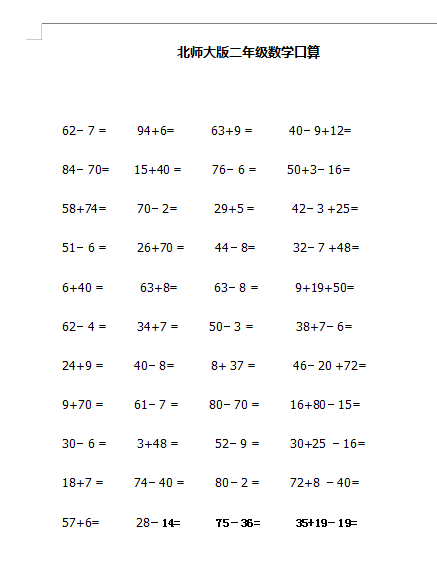62－7 =     94+6=      63+9 =      40－9+12=

84－70=    15+40 =     76－6 =     50+3－16=

58+74=     70－2=      29+5 =      42－3 +25=

51－6 =     26+70 =     44－8=      32－7 +48=

6+40 =      63+8=      63－8 =      9+19+50=

2022：新北师大版小学数学2年级下册口算 (2)（doc文档2电页子档下载）